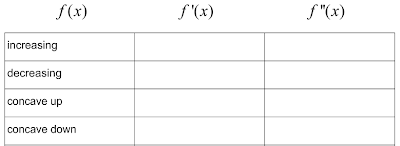## Saturday, November 23, 2013

### BFFs: f, f', and f''

In AP Calculus, we're currently working on applications of the derivative.  As I studied past AP Calc exams this summer, it was clear to me that students need a very firm understanding of the relationships between f, f', and f'' in order to be successful on the exam.  I've been gently guiding my students in this pursuit the entire semester (in fact, that's how they discovered derivatives of trig functions), but now we're diving in head first.  I know that this is not an easy concept to master.  Very few students "get" it right away (I didn't either at their age).  But, to me, that's what makes it super fun to teach.  Or try to teach.

So, here's what we have been doing in Calc AB to help students solidify these three relationships:
• Introduction to f, f', and f'' by matching their graphs in groups of 3-4 students.  The matching activity is very similar to this one.
• Students conceptualized what it means for the first derivative to be positive, but the second derivative to be negative (for example) by filling out these charts:

• Students described concisely in words through this chart:• My still all-time favorite, Inflection via Infection
• Daily Warm Up where students have to answer about ten questions like:
1. If f is increasing then f' ______________.
2. If f has a point of inflection then f' _____________.
3. If f'' is negative then f ______________.
4. If f'' is negative then f' ______________.
And then the finale:  a nine-question clicker quiz similar to the questions above.  The students who scored less than a 50% on this quick assessment are being called into lunch next week to get further help (this was totally my colleague's idea...genius!).  What I loved about the clicker quiz was that I could post the results as soon as the kids were done and then we could talk about the questions that gave them the most trouble.

For the kids who are coming in for extra help, we have created a packet where they will be given a function and then instructed to graph the function and its first two derivatives.  Then they'll answer questions like "Where is f concave up?"  "Where is f'' positive?"  "Where is f' increasing?"  And, hopefully, they'll see that the answers to all three questions are the same.

It seems my students do fairly well when they are asked questions about what the first and second derivatives tell you about the original function.  However, they have a hard time telling you what the second derivative tells you about the first derivative.  They don't seem to make the connection that that's the same thing as asking what does the first derivative tell you about the original function (which, like I said, they can do just fine!).  For example, on the quiz, the first two questions were:

1. If f is increasing, then f' is ____________.
2. If f' is increasing, then f'' is ___________.
They did beautifully on the first question; horribly on the second.  When I asked them, "Do you see how the two questions are the same?  In each case, you've only derived once."  I got a few "Ah-ha!"'s, but I think several are still struggling to see the connection.  So, that led me to create this chart:

No words.  All symbols.  And I purposely did not call any of the functions f.  My hope is, if they can understand this flow chart, they will now be able to answer questions like #2 above.  We shall see how it goes.

What other things do you do to help students with these ever-important relationships?